Home > A2C > Chapter 8 > Lesson 8.1.7 > Problem8-104

8-104.
1. What central angle, measured in degrees, corresponds to a distance around the unit circle of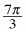? Homework Help ✎

1. What other angles will take you to the same point on the circle?

2. Make a sketch of the unit circle showing the resulting right triangle.

3. Find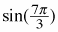,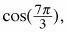and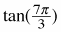exactly.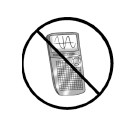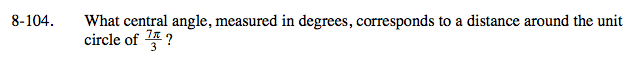$\text{What\:number\:of\:degrees\:is\:equivalent\:to}\:\frac{\pi}{3}\:\text{radians?}$

If you can't remember, calculate.

$\pi=180\degree \ \ \ \ \ \ \ \ \frac{\pi}{3} = 60^\circ$

Now multiply the angle by 7.

420°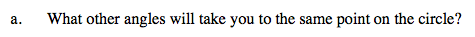The distance around the unit circle is 2π, no matter what point you start from.

$\frac{\pi}{3} \:\pm\:2\pi n$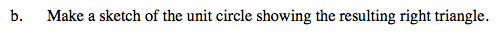The angle you are working with, 420°, is more than 360°. How much more?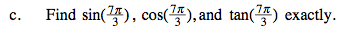Notice that the triangle formed is a 30°-60°-90° triangle.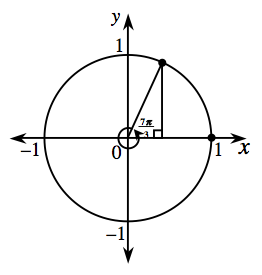$\text{sin\left(\frac{7\pi}{3}\right)=\frac{\sqrt{3}}{2}}$

$\text{cos\left(\frac{7\pi}{3}\right)=\frac{1}{2}}$

$\text{tan\left(\frac{7\pi}{3}\right)=\sqrt{3}}$